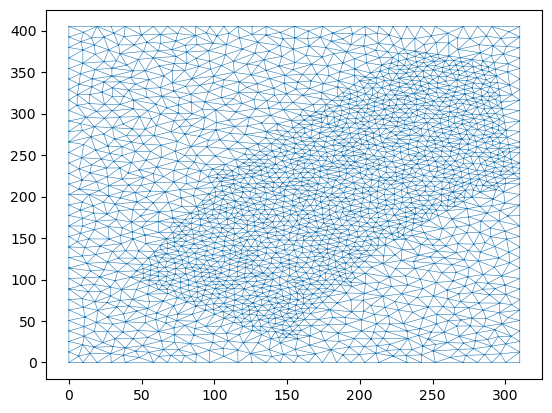# Merewether Flood Case Study Example

Here we look at a case study of a flood in the community of Merewether near Newcastle NSW. We will add a flow using an Inlet_operator and extract flow details at various points by interagating the sww file which is produced during the ANUGA run.

This example is based on the the validation test merewether, provided in the ANUGA distribution.

## Setup Notebook for Visualisation and Animation

We are using the format of a jupyter notebook. As such we need to setup inline matplotlib plotting and animation.

:

import numpy as np
import os
import matplotlib.pyplot as plt

%matplotlib inline

# Allow inline jshtml animations
from matplotlib import rc
rc('animation', html='jshtml')


## Import ANUGA

We assume that anuga has been installed. If so we can import anuga.

:

import anuga


We have included some topography data and extent data in our anuga-clinic notebook repository.

Let’s read that in and create a mesh associated with it.

:

data_dir = '/home/anuga/anuga-clinic/data/merewether'

# Polygon defining broad area of interest

# Polygon defining particular area of interest

# Elevation Data
topography_file = os.path.join(data_dir,'topography1.asc')

# Resolution for most of the mesh
base_resolution = 80.0  # m^2

# Resolution in particular area of interest
merewether_resolution = 25.0 # m^2

interior_regions = [[merewether_polygon, merewether_resolution]]


## Create and View Domain

Note that we use a base_resolution to ensure a reasonable refinement over the whole region, and we use interior_regions to refine the mesh in the area of interest. In this case we pass a list of polygon, resolution pairs.

:

domain = anuga.create_domain_from_regions(
bounding_polygon,
boundary_tags={'south': ,
'east':  ,
'north':    ,
'west':   },
maximum_triangle_area=base_resolution,
interior_regions=interior_regions)

domain.set_name('merewether1') # Name of sww file
dplotter = anuga.Domain_plotter(domain)
plt.triplot(dplotter.triang, linewidth = 0.4);

Figure files for each frame will be stored in _plot## Setup Initial Conditions

We have to setup the values of various quantities associated with the domain. In particular we need to setup the elevation the elevation of the bed or the bathymetry. In this case we will do this using the DEM file topography1.asc .

:

domain.set_quantity('elevation', filename=topography_file, location='centroids') # Use function for elevation
domain.set_quantity('friction', 0.01, location='centroids')                        # Constant friction
domain.set_quantity('stage', expression='elevation', location='centroids')         # Dry Bed

plt.tripcolor(dplotter.triang,
facecolors = dplotter.elev,
cmap='Greys_r')
plt.colorbar();
plt.title("Elevation");## Setup Boundary Conditions

The rectangular domain has 4 tagged boundaries, left, top, right and bottom. We need to set boundary conditons for each of these tagged boundaries. We can set Transmissive type BC on the outflow boundaries and reflective on the others.

:

Br = anuga.Reflective_boundary(domain)
Bt = anuga.Transmissive_boundary(domain)

domain.set_boundary({'south':   Br,
'east':    Bt, # outflow
'north':   Bt, # outflow
'west':    Br})


## Setup Inflow

We need some water to flow. The easiest way to input a specified amount of water is via an Inlet_operator where we can specify a discharge Q.

:

# Setup inlet flow
center = (382270.0, 6354285.0)
fixed_inflow = anuga.Inlet_operator(domain, region0 , Q=19.7)


## Run the Evolution

We evolve using a for statement, which evolves the quantities using the shallow water wave solver. The calculation yields every yieldstep seconds, up to a given duration.

:

for t in domain.evolve(yieldstep=20, duration=300):

#dplotter.plot_depth_frame()
dplotter.save_depth_frame(vmin=0.0, vmax=1.0)

domain.print_timestepping_statistics()

# Read in the png files stored during the evolve loop
dplotter.make_depth_animation()

Time = 0.0000 (sec), steps=0 (16s)
Time = 20.0000 (sec), delta t = 1000.00000000 (s), steps=1 (0s)
Time = 40.0000 (sec), delta t in [0.31097778, 0.54228760] (s), steps=57 (0s)
Time = 60.0000 (sec), delta t in [0.19738976, 0.34302832] (s), steps=78 (0s)
Time = 80.0000 (sec), delta t in [0.19746572, 0.20781137] (s), steps=99 (0s)
Time = 100.0000 (sec), delta t in [0.19296721, 0.21299123] (s), steps=99 (0s)
Time = 120.0000 (sec), delta t in [0.18074822, 0.19291818] (s), steps=107 (0s)
Time = 140.0000 (sec), delta t in [0.16806655, 0.18068880] (s), steps=116 (0s)
Time = 160.0000 (sec), delta t in [0.15436803, 0.16804974] (s), steps=123 (0s)
Time = 180.0000 (sec), delta t in [0.15154953, 0.15428113] (s), steps=132 (0s)
Time = 200.0000 (sec), delta t in [0.15069124, 0.15217552] (s), steps=133 (0s)
Time = 220.0000 (sec), delta t in [0.14955219, 0.15068963] (s), steps=134 (0s)
Time = 240.0000 (sec), delta t in [0.14931204, 0.14955927] (s), steps=134 (0s)
Time = 260.0000 (sec), delta t in [0.14956369, 0.14977760] (s), steps=134 (0s)
Time = 280.0000 (sec), delta t in [0.14947921, 0.14972423] (s), steps=134 (0s)
Time = 300.0000 (sec), delta t in [0.14941875, 0.14947894] (s), steps=134 (0s)

: